• 简单来说是：参数估计使用样本统计量估计总体的参数的 【百度百科的解释如下】 参数估计（parameter estimation），统计推断的一种。根据从总体中抽取的随机样本来估计总体分布中未知参数的过程。从估计形式看，...

参数估计

1、什么是参数估计

简单来说是：参数估计是指使用样本统计量估计总体的参数的
【百度百科的解释如下】
参数估计（parameter estimation），统计推断的一种。根据从总体中抽取的随机样本来估计总体分布中未知参数的过程。从估计形式看，区分为点估计与区间估计：从构造估计量的方法讲，有矩法估计、最小二乘估计、似然估计、贝叶斯估计等。要处理两个问题：（1）求出未知参数的估计量；（2）在一定信度（可靠程度）下指出所求的估计量的精度。信度一般用概率表示，如可信程度为95%；精度用估计量与被估参数（或待估参数）之间的接近程度或误差来度量。

2、为什么需要参数估计

人们常常需要根据手中的数据，分析或推断数据反映的本质规律。即根据样本数据如何选择统计量去推断总体的分布或数字特征等。统计推断是数理统计研究的核心问题。所谓统计推断是指根据样本对总体分布或分布的数字特征等作出合理的推断，也就我我们所讨论的参数估计

3、参数估计有可以分为哪几种

参数估计通常可以分为：点估计和区间估计两种

3.1点估计

点估计：在统计学中，点估计（point estimation）是指用样本数据来估计总体参数， 估计结果使用一个点的数值表示“最佳估计值”，因此称为点估计。由样本数据估计总体分布所含未知参数的真值，所得到的值，称为估计值
常用的估计法有：最小方差均值无偏估计（MVUE）、最佳线性无偏估计（BLUE）、最小均方误差（MMSE）、中值无偏估计、最大似然估计（MLE）、矩估计和广义矩估计。本文主要介绍一下最大似然估计（MLE）和矩估计。

3.1.1 最大似然估计（MLE）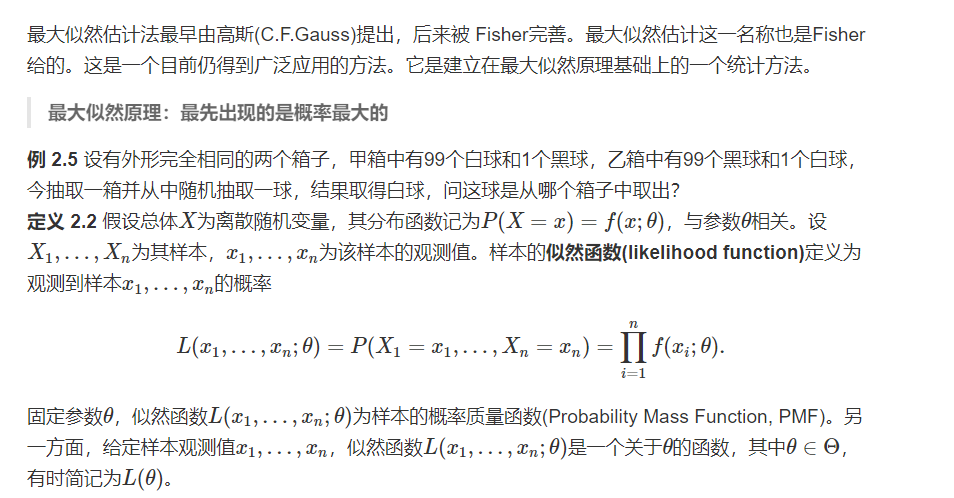3.1.2 矩估计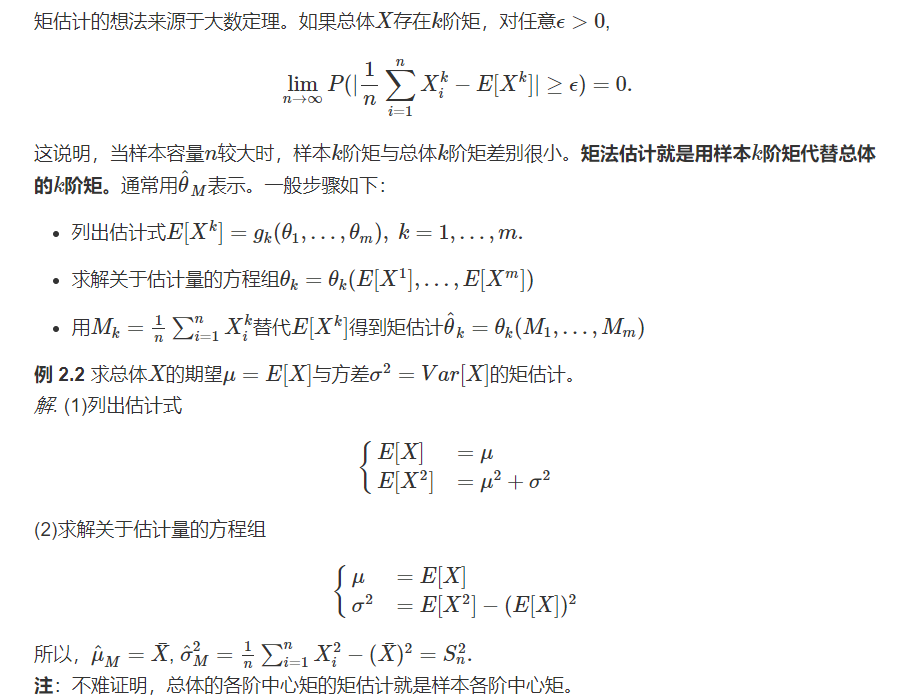3.2区间估计

区间估计：在点估计的基础上，给出总体参数的一个区间范围，通常是由样本统计量加减估计误差得到
置信区间：在区间估计中，由样本统计量构造的总体参数的估计区间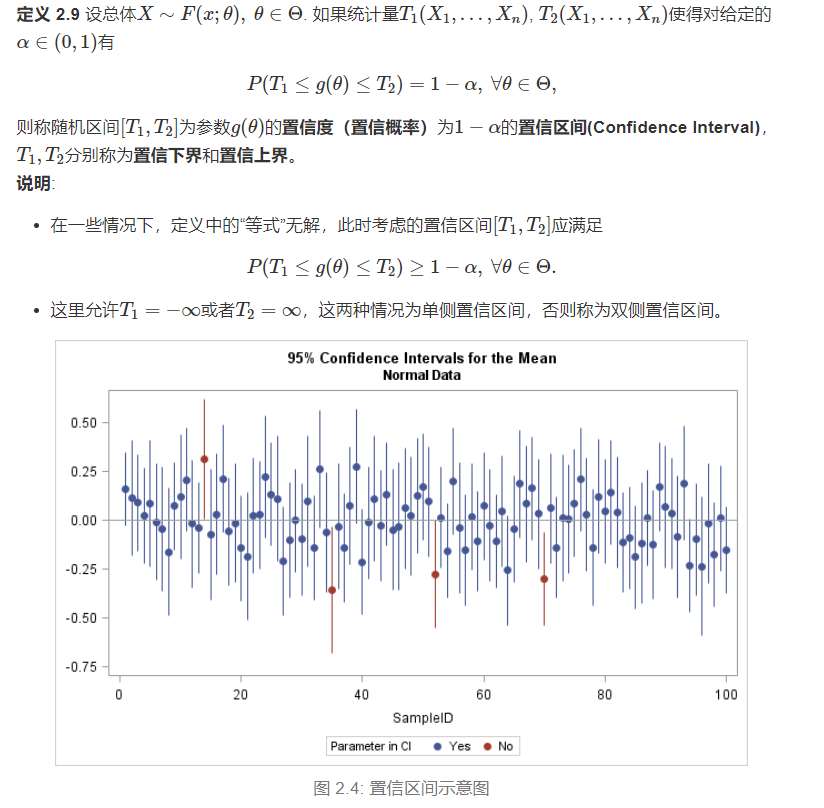参考

展开全文算法
• 什么是参数估计？

千次阅读 2020-10-20 20:06:51
参数估计(parameter estimation) 参数估计属于统计推断的范畴，是根据从总体中抽取的样本估计总体分布中包含的未知参数的方法。 统计推断是数理统计研究的核心问题，是根据样本对总体分布或分布的数字特征等作出...

参数估计(parameter estimation)

目录

参数估计(parameter estimation)

点估计（point estimation）

矩估计法（method  of  moments）,

区间估计（interval estimation）

参数估计属于统计推断的范畴，是根据从总体中抽取的样本估计总体分布中包含的未知参数的方法。
统计推断是数理统计研究的核心问题，是指根据样本对总体分布或分布的数字特征等作出合理的推断。
参数估计分为：点估计、区间估计

点估计（point estimation）

点估计是依据样本估计总体分布中所含的未知参数或未知参数的函数。通常它们是总体的某个特征值，如数学期望、方差和相关系数等。点估计问题就是要构造一个只依赖于样本的量，作为未知参数或未知参数的函数的估计值。例如，设一批产品的废品率为θ。为估计θ，从这批产品中随机地抽出n 个作检查，以X记其中的废品个数，用X/n估计θ，这就是一个点估计。

构造点估计常用方法：

• 矩估计法：用样本矩估计总体矩，比如：用样本均值估计总体均值。
• 最大似然估计法：于1912年由英国统计学家R.A.费希尔提出，利用样本分布密度构造似然函数来求出参数的最大似然估计。
• 最小二乘法：主要用于线性统计模型中的参数估计问题。比如：Y=a0+a1X的参数估计就可以用最小乘法。
• 贝叶斯估计法：基于贝叶斯学派的观点而提出的估计法。可以用来估计未知参数的估计量很多，于是产生了怎样选择一个优良估计量的问题。首先必须对优良性定出准则，这种准则是不唯一的，可以根据实际问题和理论研究的方便进行选择。优良性准则有两大类:一类是小样本准则，即在样本大小固定时的优良性准则;另一类是大样本准则，即在样本大小趋于无穷时的优良性准则。最重要的小样本优良性准则是无偏性及与此相关的一致最小方差无偏估计，其次有容许性准则， 最小化最大准则，最优同变准则等。大样本优良性准则有相合性、最优渐近正态估计和渐近有效估计等。

点估计能够明确告知人们“未知参数是多少”，但不能反映估计的可信程度。

矩估计法（method  of  moments）,

矩估计法也称"矩法估计"，原理是用样本矩作为相应的总体矩估计来求出估计量的方法，其思想是如果总体中有 K个未知参数，可以用前 K阶样本矩估计相应的前k阶总体矩，然后利用未知参数与总体矩的函数关系，求出参数的估计量。
矩法估计一般求的是一阶原点矩二阶中心矩

假设总体X的k阶原点矩：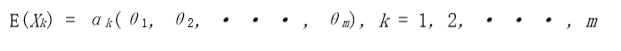令总体的k阶原点矩等于它样本的k阶原点矩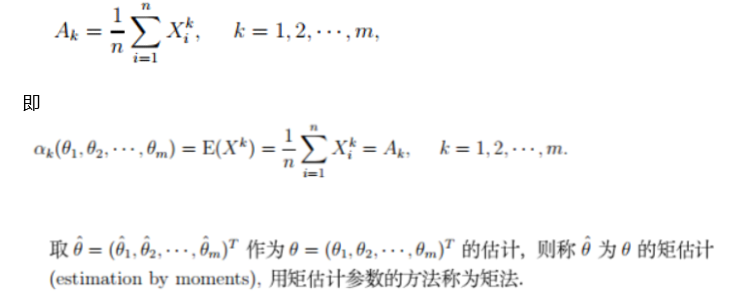注：矩法相比于极大似然法、最小二乘法，效率很低。目前很少使用。

区间估计（interval estimation）

区间估计是依据抽取的样本，根据一定的正确度与精确度的要求，构造出适当的区间，作为总体分布的未知参数或参数的函数的真值所在范围的估计。

例如人们常说的有百分之多少的把握保证某值在某个范围内，即是区间估计的最简单的应用。

求置信区间常用的三种方法:

• 利用已知的抽样分布。
• 利用区间估计与假设检验的联系。
• 利用大样本理论。

区间估计可以告知置信区间范围，但不能直接告知人们“未知参数是多少”。

置信区间

区间估计(interval estimation)是从点估计值和抽样标准误出发，按给定的概率值建立包含待估计参数的区间。其中这个给定的概率值称为置信度或置信水平(confidence level)，这个建立起来的包含待估计参数的区间称为置信区间(confidence interval)，指总体参数值落在样本统计值某一区内的概率。

所谓置信水平就是给出一个区间的信心，这个信心以概率来表示，绝大多数情况下取 0.95，表示你对所估计的总体参数有95%的信心落在你所给的区间内。通常置信水平以1-α表 示，α称为显著性水平

置信区间的建立就与中心极限定理和抽样分布有关了，在给定置信度的条件下，置信区间的宽度决定于抽样分布。 建立置信区间的意思是在设定的置信水平(如取0.95)下，总体参数落在这个区间的概率为 0.95，大致的理解是如果抽100次样，建立100个置信区间，大约95个区间包含总体参数，约5个区间不包含总体参数(注意不是一定有5个，可能会多，也可能会少)。

划定置信区间的两个数值分别称为置信下限(lower confidence limit,lcl)和置信上限(upper confidence limit,ucl)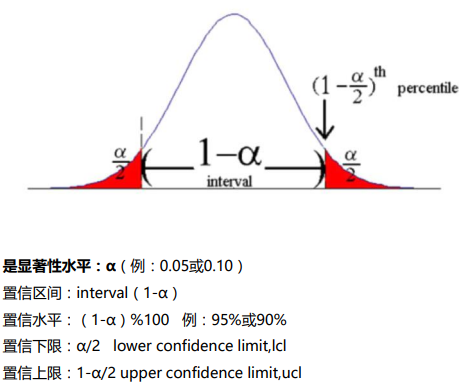置信区间最主要的应用是用于假设检验

展开全文算法 参数估计
• 极大似然估计贝叶斯估计是参数估计中的一种方法，以贝叶斯思想为基础，而贝叶斯思想在机器学习中经常用到。机器学习中常涉及贝叶斯网络，最终的问题都是转化为参数求解。贝叶斯参数估计是这些问题的基础版本。前方...

极大似然估计

贝叶斯估计是参数估计中的一种方法，以贝叶斯思想为基础，而贝叶斯思想在机器学习中经常用到。机器学习中常涉及贝叶斯网络，最终的问题都是转化为参数求解。贝叶斯参数估计是这些问题的基础版本。前方高能预警，本文的讲解比较理论。

实际问题中我们会有很多数据，比如一篇文章中每个单词的词频等。我们得到的数据通常用 X X 表示，也称为样本。我们还会假设这些数据服从某一个分布，例如最常用的正态分布，这时可以将问题表示为 X ∼ N ( μ , σ ) X \sim N(\mu, \sigma) μ \mu σ \sigma 表示正态分布的两个参数。如果这两个参数已知，这个分布就确定了，从而可以知道数据 X X 的许多性质。这种情况下，参数估计就是要估计 μ \mu s i g m a sigma 的值。最常用的参数估计方法是极大似然（或最大似然估计）估计。

一般的最大似然法求解两个参数的基本步骤是：

• 假设每个样本 X i X_i 是独立同分布（iid）的，即每一个样本都有 X i ∼ N ( μ , σ ) X_i \sim N(\mu, \sigma) .
• 求所有样本 X X 的联合分布
因为是iid，所以 X X 的联合分布等于每个样本 X i X_i 的概率密度函数的乘积，即：
L ( μ , σ 2 ; x ) = f ( x ) = ( 1 2 π σ 2 ) n exp ⁡ { − ∑ i = 1 n ( x i − μ ) 2 2 σ 2 } L(\mu, \sigma^2; \boldsymbol{x}) = f(\boldsymbol{x}) = \left(\frac{1}{\sqrt{2\pi\sigma^2}}\right)^n \exp\left\{-\sum_{i=1}^n \frac{(x_i - \mu)^2}{2\sigma^2}\right\}
• 对上述联合分布的概率密度函数取对数，即：
ℓ ( μ , σ 2 ; x ) = log ⁡ L ( μ , σ 2 ; x ) = − n 2 log ⁡ ( 2 π σ 2 ) − ∑ i = 1 n ( x i − μ ) 2 2 σ 2 \ell(\mu, \sigma^2; \boldsymbol{x}) = \log L(\mu, \sigma^2; \boldsymbol{x}) = -\frac{n}{2}\log\left(2\pi\sigma^2\right) -\sum_{i=1}^n \frac{(x_i - \mu)^2}{2\sigma^2}
• 对上述函数分别求 ∂ ℓ ∂ μ \frac{\partial \ell}{\partial \mu} ∂ ℓ ∂ σ 2 \frac{\partial \ell}{\partial \sigma^2} ，并令它们等于0，进而求得极值
• 分别对 μ \mu σ 2 \sigma^2 求二阶偏导，验证极值是最大值

上述步骤是极大似然法的求解步骤，用到的信息都是已知样本的信息。但是通常在估计参数时我们可能已经对参数有了一个大概的了解，比如已经知道 μ \mu σ 2 \sigma^2 的取值范围。仅仅知道取值范围还太简单，有时会更进一步假设 μ \mu σ 2 \sigma^2 的取值服从某个分布，这样问题就变成了在正态分布中，要估计期望 μ \mu 和方差 σ 2 \sigma^2 的值，但与极大似然法不同的是，我们事先已经知道了 μ \mu σ 2 \sigma^2 的取值是服从某种分布的，这个信息如果不用到参数估计中有点浪费了，于是问题变成：如何将这两个参数的分布结合到参数估计过程中？ 贝叶斯估计解决了这个问题。

贝叶斯估计（Bayes Estimation）

上述提到的在估计参数之前对参数已经有了了解称为参数的先验知识。贝叶斯估计即在估计过程中将先验知识也考虑了进去，博众家之长总是好的。先验知识可以是一个具体的值，也可以是取值范围，也可以是一个函数（某种分布）。实际应用中，通常会将参数的先验知识视作一个分布，那么这个参数就会有一个概率密度函数（pdf），这个pdf叫做待估计参数的先验概率

一般待估计的一维参数用 θ \theta 表示，多维用粗体 θ \boldsymbol{\theta} . 先验概率密度函数用符号 π ( θ ) \pi(\theta) 表示。样本的概率密度函数用 f ( x ∣ θ ) f(x|\theta) 表示，其中加入 θ \theta 是表示该pdf跟 θ \theta 有关，同时说明要估计的参数是 θ \theta .

参数估计必然会涉及到『损失』的概念，用于表示参数的估计值与真实值之间的差距。差距越小，损失越小，说明参数估计得约准确。

贝叶斯估计涉及到三个损失概念，他们长的很像：

• 损失函数（Loss Funcition）
• 风险函数（Risk Function）
• 贝叶斯风险（Bayes Risk）

贝叶斯估计的目的是：结合参数的先验知识，使得参数的估计值令贝叶斯风险达到最小。简单说就是最小化贝叶斯风险

下面解释这三个概念。

损失函数

在参数估计问题中，评价估计的好坏就是看估计出来的参数与真值的差距有多小。估计出来的参数通常用 θ ^ \hat{\theta} 表示，参数的真值用 θ \theta 表示。 θ \theta θ ^ \hat{\theta} 的差距就是损失函数。

损失函数有好几种定义方式，常见的如下：

• L ( θ ^ , θ ) = ( θ ^ − θ ) 2 L(\hat{\theta}, \theta) = (\hat{\theta} - \theta)^2
• L ( θ ^ , θ ) = ∣ θ ^ − θ ∣ L(\hat{\theta}, \theta) = |\hat{\theta} - \theta|
• L ( θ ^ , θ ) = { 0 if  ∣ θ − θ ^ ∣ ⩽ Δ 1 if  ∣ θ − θ ^ ∣ > Δ \begin{aligned} L(\hat{\theta}, \theta) = \begin{cases} 0 &\text{if $|\theta - \hat{\theta}| \leqslant \Delta$} \\ 1 &\text{if $|\theta - \hat{\theta}| > \Delta$} \end{cases} \end{aligned}

当估计值与真实值无限接近时，损失函数都会无限接近0，相当于没有损失。损失函数中的估计值 θ ^ \hat{\theta} 是通过样本计算出来的。比如正态分布中的 μ \mu ，我们可以用样本均值来估计 μ \mu （即将样本均值当成 μ \mu ），即 μ ^ = 1 n ∑ n X i = X ˉ \hat{\mu} = \frac{1}{n}\sum^n X_i = \bar{\boldsymbol{X}} 。类似地，也常用样本方差来估计 σ 2 \sigma^2 . 但是注意到 μ ^ \hat{\mu} σ ^ 2 \hat{\sigma}^2 的值都跟样本的个数有关，即都跟 n n 有关。如果 1 n ∑ i = 0 n X i \frac{1}{n}\sum_{i=0}^n X_i μ \mu 的估计值，那 1 n − 1 ∑ i = 0 n − 1 X i \frac{1}{n-1}\sum_{i=0}^{n-1} X_i μ \mu 的估计值吗？那 1 n − 6 ∑ i = 0 n − 6 X i \frac{1}{n-6}\sum_{i=0}^{n-6} X_i 呢？可以看到 n n 不一样，估计值也不一样，到底用哪个 n n （用多少个样本）来计算损失函数呢？这时容易（其实不容易）想到，既然损失函数可以因为 θ ^ \hat{\theta} 的不同而有不同的值，那就求平均。通过判断损失的平均值的大小来判断参数估计得好不好。求平均是很自然的想法，但平均通常是相对样本来说的，如果是总体，我们通常说期望。这时就要引入风险函数了。

风险函数

风险就是损失函数关于 θ ^ \hat{\theta} 的期望

既然估计值 θ ^ \hat{\theta} 是随 n n 的变化而变化的，那也可以将 θ ^ \hat{\theta} 视为随机变量，进而可以计算损失函数的期望。于是风险函数的定义如下：

R ( θ ^ , θ ) = E θ ^ [ L ( θ ^ , θ ) ] R(\hat{\theta}, \theta) = E_{\hat{\theta}} \left[L(\hat{\theta}, \theta) \right]

E θ ^ E_{\hat{\theta}} 表示对 θ ^ \hat{\theta} 求期望（注意真值 μ \mu 是固定的，不是变量）。我们的目标是：求出一个 θ ^ \hat{\theta} ，使得风险最小（最小化风险）

例如，要估计期望 μ \mu ，假设损失函数是 L ( μ ^ , μ ) = ( μ ^ − μ ) 2 L(\hat{\mu}, \mu) = (\hat{\mu} - \mu)^2 ，如果用样本均值作为 μ \mu 的估计值（即 μ ^ = 1 n ∑ i = 0 n X i \hat{\mu} = \frac{1}{n}\sum_{i=0}^n X_i ），这样的风险是多少？

E [ L ( μ ^ , μ ) ] = E ( X ˉ − μ ) 2 = E X ˉ 2 − 2 μ E X ˉ + μ 2    E \left[L(\hat{\mu}, \mu) \right] = E(\bar{\boldsymbol{X}} - \mu)^2 = E\bar{\boldsymbol{X}}^2 - 2\mu E\bar{\boldsymbol{X}} + \mu^2 \ \

算出上式中的 E X ˉ 2 E\bar{\boldsymbol{X}}^2 E X ˉ E\bar{\boldsymbol{X}} 就可以知道风险是多少。其实上式的风险是0，已经是最小值了。这个结果说明当使用样本均值来估计总体期望时，风险最低，所以一般都用样本均值来代替总体期望。

到目前为止并没有用到前面提过的先验知识 π ( θ ) \pi(\theta) ，仅仅定义了损失函数和风险函数。上式的风险函数求出了一个具体的值，但很多情况没有那么简单，求出的风险是一个函数表达式，而不是值。如果求出来的风险是一个值，其实可以不用贝叶斯估计（上面也一直没有用到 θ \theta 的任何先验知识）。贝叶斯估计通常用于风险不可以直接比较的情况。

这时就要引入贝叶斯风险的概念了。贝叶斯风险中就用到了 θ \theta 的先验分布 π ( θ ) \pi(\theta) .

贝叶斯风险

注意到风险函数 R ( θ ^ , θ ) R(\hat{\theta}, \theta) θ ^ \hat{\theta} 的函数，前面也提到真值 θ \theta 是一个固定的值，不是变量。但是，我们会在估计 θ \theta 之前知道一些 θ \theta 的知识，比如说 θ \theta 的分布 π ( θ ) \pi(\theta) （这个分布是怎么知道的呢？下文有讲解）。既然 θ \theta 是有一个概率分布的，那么此时 θ \theta 也变成一个随机变量了，所以 R ( θ ^ , θ ) R(\hat{\theta}, \theta) 同时是 θ ^ \hat{\theta} θ \theta 的函数。 这时要怎么使用 π ( θ ) \pi(\theta) 呢？下面定义贝叶斯风险。

贝叶斯风险：

B a y e s   R i s k = E θ [ R ( θ , θ ^ ) ] = ∫ R ( θ , θ ^ ) π ( θ ) d θ   (期望的定义) Bayes\ Risk = E_{\theta} \left[R(\theta, \hat{\theta})\right] = \int R(\theta, \hat{\theta})\pi(\theta) d\theta \text{\ (期望的定义)}

上式表示：贝叶斯风险是风险函数在 θ \theta 上的期望

回顾一下期望的定义。如果随机变量用 X \boldsymbol{X} 表示，其概率密度函数是 f ( x ) f(\boldsymbol{x}) ，那么 X \boldsymbol{X} 的期望 E X = ∫ x f ( x ) d x E\boldsymbol{X} = \int xf(x)dx . 对照期望的积分形式，可以看到 π ( θ ) \pi(\theta) 就是 θ \theta 的概率密度函数，随机变量是 θ \theta . R ( θ , θ ^ ) R(\theta, \hat{\theta}) 是关于随机变量 θ \theta 的一个函数，所以Bayes Risk就是在求风险函数的期望。总的来说：风险函数是损失函数关于 θ ^ \hat{\theta} 的期望，而贝叶斯风险是风险函数关于 θ \theta 的期望。 所以贝叶斯风险是一个双期望。更进一步， θ ^ \hat{\theta} 是关于随机变量 X X 的函数（ θ ^ \hat{\theta} 总是通过样本 X \boldsymbol{X} 求出来），所以也可以说：风险函数是损失函数关于 x \boldsymbol{x} 的期望。这样就将风险与 θ \theta 的先验知识关联起来了。

之所以叫贝叶斯风险是因为引入了一个先验分布 π ( θ ) \pi(\theta) . 『先验』这个词本身就是贝叶斯理论的一部分。

如何最小化贝叶斯风险（贝叶斯估计）

终于到达贝叶斯估计的核心了，即如何找到一个 θ ^ \hat{\theta} ，使得贝叶斯风险最小。问题转化为求 ∫ R ( θ , θ ^ ) π ( θ ) d θ \int R(\theta, \hat{\theta})\pi(\theta) d\theta 的最小值。

这是一个积分形式的函数，如果知道 R ( θ , θ ^ ) R(\theta, \hat{\theta}) π ( θ ) \pi(\theta) 的具体形式可以直接求出最小值。不过如果仅仅根据这个抽象的形式，也可以求出最小值的形式。

下面给出最小化贝叶斯风险的推导过程，不想看推导过程可以直接看最后结论。

为了跟一些书的符号统一，下面引入一个新的符号： a = θ ^ a = \hat{\theta} . 即用字母 a a 来表示参数的估计值 θ ^ \hat{\theta} .

字母 a a 表示action，表示采取某一动作求得 θ \theta 的估计。在参数估计问题中， a a 就是 θ ^ \hat{\theta} . 所以 R ( θ , θ ^ ) = R ( θ , a ) R(\theta, \hat{\theta}) = R(\theta, a)

因为 R ( θ , a ) R(\theta, a) 的本质也是求期望，先将其转换为积分的形式。

R ( θ , a ) = E a [ L ( θ , a ) ] = ∫ L ( θ , a ( x ) ) f ( x ∣ θ ) d x R(\theta, a) = E_a\left[L(\theta, a)\right] = \int L(\theta, a(x))f(x|\theta) dx

因为 a a x x 的函数，所以对 a a 求期望就是在 x x 上求期望，进而转换为对 x x 求积分。同时 x x 的概率密度函数是已知的，记为 f ( x ∣ θ ) f(x|\theta) ，表示 x x 的pdf跟 θ \theta 有关，也表示条件概率密度函数

既然在 θ \theta 给定的条件下， x x 的条件pdf是 f ( x ∣ θ ) f(x|\theta) （此时已经将 θ \theta 当成随机变量看待了），而且 θ \theta 的分布又是 π ( θ ) \pi(\theta) ，那么根据条件概率的定义，可以求出 x x 的边缘概率密度函数：

f ( x ) = ∫ f ( x ∣ θ ) π ( θ )   d θ   (边缘概率定义) f(x) = \int f(x|\theta) \pi(\theta)\ d\theta \ \ \text{(边缘概率定义)}
所以有：

∫ R ( θ , a ) π ( θ ) d θ = ∫ θ [ ∫ x L ( θ , a ( x ) )   f ( x ∣ θ )   d x ] π ( θ )   d θ (带入上式展开) = ∫ θ ∫ x L ( θ , a ( x ) ) f ( x ∣ θ ) π ( θ ) f ( x ) f ( x )   d x   d θ   (除以一个 f ( x ) 再乘以一个 f ( x ) 结果不变) = ∫ θ ∫ x L ( θ , a ( x ) ) π ( θ ∣ x )   f ( x )   d x   d θ  (贝叶斯定理) = ∫ x [ ∫ θ L ( θ , a ( x ) ) π ( θ ∣ x )   d θ ] f ( x )   d x    (交换积分顺序) \begin{aligned} \int R(\theta, a)\pi(\theta) d\theta &= \int_{\theta} \left[\int_x L(\theta, a(x))\ f(x|\theta)\ dx\right] \pi(\theta)\ d\theta & \text{(带入上式展开)}\\ &= \int_{\theta} \int_x L(\theta, a(x)) \frac{f(x|\theta) \pi(\theta)}{f(x)} f(x)\ dx\ d\theta & \ \text{(除以一个$f(x)$再乘以一个$f(x)$结果不变)} \\ &= \int_{\theta} \int_x L(\theta, a(x)) \pi(\theta|x)\ f(x)\ dx\ d\theta & \ \text{(贝叶斯定理)} \\ &= \int_x \left[\int_{\theta} L(\theta, a(x)) \pi(\theta|x)\ d\theta \right] f(x)\ dx\ & \ \text{(交换积分顺序)} \\ \end{aligned}

最后一步无法化简了，那就分析上式中最后一项。

通过贝叶斯定理，求出了 π ( θ ∣ x ) \pi(\theta|x) . 观察最后一项中括号中的式子， π ( θ ∣ x ) \pi(\theta|x) 是一个pdf，中括号中的这一项很像期望的定义。实际上中括号这一项就是 π ( θ ∣ x ) \pi(\theta|x) 上求损失函数 L ( θ , a ( x ) ) L(\theta, a(x)) 的期望。

回顾贝叶斯估计的目的：求出 θ ^ \hat{\theta} （在这里是 a ( x ) a(x) ），使得贝叶斯风险最小（即 ∫ R ( θ , a ) π ( θ ) d θ \int R(\theta, a)\pi(\theta) d\theta 最小）

中括号中的那一项是对 θ \theta 积分，积分后不会有 θ \theta ，从而整个式子只剩下 x x . 而回想一下贝叶斯估计的目的， x x 并不是我们要关心的。所以贝叶斯估计就是要计算中括号一项，使得中括号内的积分最小，最终还是回到了损失函数上。

上面提到中括号一项看起来像是期望的定义，其实这一项称为posterior expected risk. 记作：

∫ θ L ( θ , a ( x ) ) π ( θ ∣ x )   d θ = E π L ( θ , a ( x ) ) \int_{\theta} L(\theta, a(x)) \pi(\theta|x)\ d\theta = E_{\pi} L(\theta, a(x))

E π E_{\pi} 表示在 π ( θ ∣ x ) \pi(\theta|x) 上求期望。 π ( θ ∣ x ) \pi(\theta|x) 就叫做 θ \theta 的后验分布，即在知道数据 x x θ \theta 的分布。所以贝叶斯估计就是： θ ^ \hat{\theta} ，使得损失函数在 θ \theta 的后验分布上的期望最小。

此时可以发现， 损 失 函 数 L ( θ , a ( x ) ) 损失函数L(\theta, a(x)) 是自己设计的，比如前面提到的那三种。如果知道 π ( θ ) \pi(\theta) ，根据贝叶斯定理，容易求出 π ( θ ∣ x ) \pi(\theta|x) ；而 π ( θ ) \pi(\theta) 也是我们自己定义的（先验知识，肯定是事先就知道了的，不知道也可以假设），所以这个posterior expected risk不难求得。至于如何计算后验分布，后面有讲解。

结论

贝叶斯参数估计的步骤：

• 拿到数据，知道数据的分布（或者定义数据的分布），记为 f ( x ∣ θ ) f(\boldsymbol{x}|\theta) ，要估计的参数记为 θ \theta
• 定义损失函数 L ( θ , θ ^ ) L(\theta, \hat{\theta})
• 定义（或者假设） θ \theta 的先验知识或先验分布 π ( θ ) \pi(\theta)
• 根据贝叶斯定理求出后验分布 π ( θ ∣ x ) = f ( x ∣ θ ) π ( θ ) f ( x ) \pi(\theta|\boldsymbol{x}) = \frac{f(\boldsymbol{x}|\theta)\pi(\theta)}{f(\boldsymbol{x})}
• 最小化如下式子：
arg ⁡ min ⁡ ∫ L ( θ , θ ^ ) π ( θ ∣ x )   d θ = arg ⁡ min ⁡ E π L ( θ , θ ^ ) \arg \min \int L(\theta, \hat{\theta}) \pi(\theta|\boldsymbol{x})\ d\theta = \arg \min E_{\pi} L(\theta, \hat{\theta})

上面最后一步，涉及积分以及最小值求解，看起来十分麻烦。所幸，在特定的损失函数形式下，上面最后一步可以化简，并不需要完全用到积分。下面讲解在三种特定损失函数形式下贝叶斯估计的计算方法。

理论总是简单的，上述的求解过程后面附有例子，可以温习一下。

三种常用损失函数的贝叶斯估计计算

Square Error

square error就是

L ( θ , a ) = ( θ − a ) 2 L(\theta, a) = (\theta - a)^2

下面这直接给出结论：

若损失函数是square error，那么当 a a 等于 θ \theta π ( θ ∣ x ) \pi(\theta|x) 上的期望时，贝叶斯风险最小。

上述结论证明涉及到其它知识，略过。

上述结论说明，如果知道 π ( θ ∣ x ) \pi(\theta|x) 的形式，那么只需求 ∫ θ π ( θ ∣ x )   d θ \int\theta\pi(\theta|x)\ d\theta 就可以了，实际上就是期望。例如，如果 π ( θ ∣ x ) \pi(\theta|x) 是正态分布， π ( θ ∣ x ) ∼ N ( η , τ ) \pi(\theta|x) \sim N(\eta, \tau) ，那么 a = η a = \eta η \eta 就是参数 θ \theta 的估计。

Absolute Error

Absolute error定义如下：

L ( θ ^ , θ ) = ∣ θ ^ − θ ∣ L(\hat{\theta}, \theta) = |\hat{\theta} - \theta|

下面直接给出结论：

若损失函数是square error，那么当 a a 等于数据 X X 的中位数时，贝叶斯风险最小。

这个结论说明：如果使用square error作为损失函数，不需要定义 θ \theta 的后验分布，直接取中位数。

Uniform Error

uniform error的形式为：

L ( θ ^ , θ ) = { 0 if  ∣ θ − θ ^ ∣ ⩽ Δ 1 if  ∣ θ − θ ^ ∣ > Δ \begin{aligned} L(\hat{\theta}, \theta) = \begin{cases} 0 &\text{if $|\theta - \hat{\theta}| \leqslant \Delta$} \\ 1 &\text{if $|\theta - \hat{\theta}| > \Delta$} \end{cases} \end{aligned}

下面直接给出结论：

若损失函数是uniform error且 Δ \Delta 很小，当 a = arg ⁡ max ⁡ π ( θ ∣ x ) a = \arg \max \pi(\theta|x) 时，即 a a 等于 θ \theta 后验分布的最大值时，贝叶斯风险最小。

上述结论说明，在uniform error的情况下，如果知道 π ( θ ) \pi(\theta) 的形式，那么求它的最大值即可。例如，如果 π ( θ ∣ x ) \pi(\theta|x) 是正态分布， π ( θ ∣ x ) ∼ N ( η , τ ) \pi(\theta|x) \sim N(\eta, \tau) ，正态分布的最大值在均值处取得，所以 θ \theta 的估计值为 η \eta ，与square error一样。

如何确定先验分布（先验知识）与后验分布

前面一直提到先验知识或者先验分布，偶尔混用。这是因为 θ \theta 的先验知识 π ( θ ) \pi(\theta) 可以有很多种形式，可以是一个数，可以是离散的几个数，也可以是个概率分布函数，此时称为后验分布。

但是对于后验分布，它只能是一个概率分布形式，即 π ( θ ∣ x ) \pi(\theta|x) 必须满足概率密度函数的定义，而 π ( θ ) \pi(\theta) 却不一定。原因是最后求贝叶斯风险最小值的函数形式只涉及到 π ( θ ∣ x ) \pi(\theta|x) ，没有涉及到 π ( θ ) \pi(\theta) ，所以 π ( θ ) \pi(\theta) 取什么值在数学上无所谓，但是会对结果造成影响。

很多应用中 π ( θ ) \pi(\theta) 会取一个概率密度函数。下面介绍一种 π ( θ ) \pi(\theta) 的取法：共轭先验

共轭先验（Conjugate Prior）

共轭先验这四个字指的不是一个分布，而是指一大类分布，比如指数族分布。下面给出共轭分布不太严谨的数学定义：

设资料 X X 有概率密度函数 F F X ∼ F ( x ∣ θ ) X \sim F(x| \theta) . θ \theta 的先验分布 π ( θ ) \pi(\theta) 属于某个分布族 P P π ( θ ) ∈ P \pi(\theta) \in P . 如果对任意 θ \theta θ \theta 的后验分布 π ( θ ∣ x ) \pi(\theta|x) 也属于分布族 P P ，那么 P P 就叫做 F F 的共轭先验。

白话解释：

如果找到一个 π ( θ ) \pi(\theta) ，它是 F F 的共轭先验，那么 θ \theta 的后验分布 π ( θ ∣ x ) \pi(\theta|x) 和先验分布 π ( θ ) \pi(\theta) 会有一样的形式，即同属于分布族 P P 。注意共轭是指 π ( θ ) \pi(\theta) f ( x ∣ θ ) f(x|\theta) 共轭。

『轭』是指驾车时套在牲口脖子上的曲木。古代拉扯的牲口通常有两只，因此轭是连接两只牲口的工具。在这里共轭是指 π ( θ ) π(\theta) π ( θ ∣ x ) π(\theta|x) 通过 f ( x ∣ θ ) f(x|\theta) 联系起来了。

前面说到贝叶斯估计最终需要计算出 θ \theta 的后验分布， θ \theta 的先验分布 π ( θ ) \pi(\theta) 分布是已知的。如果 π ( θ ) \pi(\theta) f ( x ) f(x) 共轭，那么 π ( θ ) \pi(\theta) π ( θ ∣ x ) \pi(\theta|x) 会有一样的形式，这样不就会很方便求解 π ( θ ∣ x ) \pi(\theta|x) ？事实上就是这样的，因此在设计先验分布的时候常常会设计成与 f ( x ) f(x) 共轭，后面计算会方便。

仅根据定义凭空想出一个共轭先验比较难。幸运的是可以证明，所有属于指数族分布的 f ( x ) f(x) ，都可以求出它的共轭先验分布 π ( θ ) \pi(\theta) 的具体形式。而大部分常见的分布都属于指数族分布（Exponential Family），比如正态分布，指数分布，二项分布，泊松分布，Beta分布，Gamma分布等等。

下面给出常见的共轭先验：如果样本的分布是上面表中第二列中的一项，那么就可以将先验分布设计成第三列中对应的分布。所以在才会在那么多算法或实际问题中将某个参数的分布定义成Gamma或Beta这种『奇怪』的形式，原因之一是为了求解方便，而且符合贝叶斯参数估计的数学原理。

后验分布的计算

贝叶斯估计的落脚点之一是求解参数的后验分布 π ( θ ∣ x ) \pi(\theta|x) . 本部分讲解求解 π ( θ ∣ x ) \pi(\theta|x) 的步骤。

先给出公式：
π ( θ ∣ x ) = f ( x ∣ θ ) π ( θ ) f ( x ) \pi(\theta|x) = \frac{f(x|\theta)\pi(\theta)}{f(x)}

贝叶斯理论中是将参数作为随机变量看待的，因此样本的概率密度函数通常写成 f ( x ∣ θ ) f(x|\theta) 的形式，表示 θ \theta 是已知的，而 f ( x ) f(x) 就是 x x 的边缘密度概率函数，这个需要计算。

泊松分布的例子

这个例子很理论，但胜于比较典型。

问题：已知 X 1 , X 2 , . . . , X n X_1, X_2, ..., X_n 是iid的，服从泊松分布， X i ∼ P o i ( λ ) X_i \sim Poi(\lambda) . λ \lambda 是要估计的参数。

（1） 利用 λ \lambda 的共轭先验求 λ \lambda 的后验分布
（2） 求在square error下 λ \lambda 的贝叶斯估计 λ ^ b a y e s \hat{\lambda}_{bayes}

求解（1）

依题意，写出 X i X_i 的概率密度函数：
f ( x ∣ λ ) = e − λ λ x x ! f(x|\lambda) = \frac{e^{-\lambda}\lambda^x}{x!}

因为 X i X_i 是独立同分布，所以它的联合概率密度函数是：

f ( x ∣ λ ) = e − n λ λ ∑ i = 1 n x i ∏ i = 1 n ( x i ! )  (连乘) f(\boldsymbol{x}|\lambda) = \frac{e^{-n\lambda}\lambda^{\sum_{i=1}^n x_i}}{\prod_{i=1}^n (x_i!)} \ \text{(连乘)}

由之前的表格可知，泊松分布的共轭先验分布是Gamma分布，Gamma分布的参数是 α \alpha β \beta ，这两个参数可以当成是已知的。所以先验分布可以写成：

π ( λ ) = β α λ α − 1 e − λ β Γ ( α ) \pi(\lambda) = \frac{\beta^\alpha \lambda^{\alpha-1} e^{-\lambda \beta}}{\Gamma(\alpha)}

求解 X X 的边缘概率密度函数 f ( x ) f(x) （下面积分过程比较简单，但太碍眼，可以略过不看）

f ( x ) = ∫ 0 ∞ f ( x ∣ λ ) π ( λ ) d λ  （连续函数需要积分，如果是离散就就求和） = ∫ 0 ∞ e − n λ λ ∑ i = 1 n x i ∏ i = 1 n ( x i ! ) β α λ α − 1 e − λ β Γ ( α ) d λ = β α ∏ i = 1 n ( x i ! ) Γ ( α ) ∫ 0 ∞ λ ∑ i = 1 n x i + α − 1 e ( n + β ) λ d λ = ( 1 n + β ) ∑ i = 1 n x i + α β α ∏ i = 1 n ( x i ! ) Γ ( α ) ∫ 0 ∞ ( ( n + β ) λ ) ( ∑ i = 1 n x i + α ) − 1 e ( n + β ) λ d ( n + β ) λ = β α ∏ i = 1 n ( x i ! ) Γ ( α ) Γ ( ∑ i = 1 n x i + α ) ( 1 n + β ) ∑ i = 1 n x i + α \begin{aligned} f(\boldsymbol{x}) &= \int_0^\infty f(\boldsymbol{x}|\lambda) \pi(\lambda) d\lambda\ \text{（连续函数需要积分，如果是离散就就求和）}\\ &= \int_0^\infty\frac{e^{-n\lambda}\lambda^{\sum_{i=1}^n x_i}}{\prod_{i=1}^n (x_i!)} \frac{\beta^\alpha \lambda^{\alpha-1} e^{-\lambda \beta}}{\Gamma(\alpha)} d\lambda \\ &= \frac{\beta^\alpha}{\prod_{i=1}^n (x_i!) \Gamma(\alpha)} \int_0^\infty \frac{\lambda^{\sum_{i=1}^n x_i + \alpha - 1}}{e^{(n+\beta)\lambda}} d\lambda \\ &= \left(\frac{1}{n+\beta}\right)^{\sum_{i=1}^n x_i + \alpha} \frac{\beta^\alpha}{\prod_{i=1}^n (x_i!) \Gamma(\alpha)} \int_0^\infty \frac{\left((n+\beta)\lambda\right)^{(\sum_{i=1}^n x_i + \alpha) - 1}}{e^{(n+\beta)\lambda}} d(n+\beta)\lambda \\ &= \frac{\beta^\alpha}{\prod_{i=1}^n (x_i!) \Gamma(\alpha)} \Gamma(\sum_{i=1}^n x_i + \alpha) \left(\frac{1}{n+\beta}\right)^{\sum_{i=1}^n x_i + \alpha} \end{aligned}

根据贝叶斯定理求解 λ \lambda 的后验分布：

π ( λ ∣ x ) = f ( x ∣ λ ) π ( λ ) f ( x ) = e − n λ λ ∑ i = 1 n x i ∏ i = 1 n ( x i ! ) β α λ α − 1 e − λ β Γ ( α ) ∏ i = 1 n ( x i ! ) Γ ( α ) ( n + β ) ∑ i x i + α β α Γ ( ∑ i = 1 n x i + α ) = e − ( n + β ) λ λ ∑ i x i + α − 1 ( n + β ) ∑ i x i + α Γ ( ∑ i = 1 n x i + α ) \begin{aligned} \pi(\lambda|\boldsymbol{x}) &= \frac{f(\boldsymbol{x}|\lambda) \pi(\lambda)}{f(\boldsymbol{x})} \\ &= \frac{e^{-n\lambda}\lambda^{\sum_{i=1}^n x_i}}{\prod_{i=1}^n (x_i!)} \frac{\beta^\alpha \lambda^{\alpha-1} e^{-\lambda \beta}}{\Gamma(\alpha)} \frac{\prod_{i=1}^n (x_i!) \Gamma(\alpha) (n+\beta)^{\sum_i x_i + \alpha}}{\beta^\alpha \Gamma(\sum_{i=1}^n x_i + \alpha) } \\ &= \frac{e^{-(n+\beta)\lambda} \lambda^{\sum_i x_i + \alpha - 1} (n+ \beta)^{\sum_i x_i + \alpha}}{\Gamma(\sum_{i=1}^n x_i + \alpha)} \end{aligned}

上面的式子很复杂，但其实它是一个Gamma分布：
π ( λ ∣ x ) ∼ Γ ( ∑ i = 1 n x i + α , n + β ) \pi(\lambda|\boldsymbol{x}) \sim \Gamma\left(\sum_{i=1}^n x_i + \alpha, n+\beta \right)

上面的求解过程还是太复杂，其实有更简便的方法。因为共轭先验分布是Gamma分布，所以后验分布肯定也是Gamma，我们可以直接凑出后验分布的形式，但不是很直观，略过。

求解（2）
Square error下的贝叶斯估计就是后验分布的期望。对于 X ∼ Γ ( α , β ) X \sim \Gamma( \alpha, \beta ) E X = α β EX = \frac{\alpha}{\beta} . 所以对于本问题：
λ ^ b a y e s = ∑ i = 1 n x i + α n + β \hat{\lambda}_{bayes} = \frac{\sum_{i=1}^n x_i + \alpha}{n+\beta}

可以看到先验分布中 λ \lambda 服从参数为 α \alpha β \beta 的Gamma分布，在观察到一些数据后， λ \lambda 仍然是服从Gamma分布的，只不过参数得到了修正，变成了 ∑ i = 1 n x i + α \sum_{i=1}^n x_i + \alpha n + β n+\beta . 这就是贝叶斯估计的思想，先假设参数服从某个分布，可能会有偏差。有偏差不要紧，我们将观察到的数据（样本）带入贝叶斯估计的过程便可以修正这些偏差， α \alpha β 就 是 修 正 因 子 \beta就是修正因子

二项分布的例子（点击率的贝叶斯平滑）

问题：已知 X 1 , X 2 , . . . , X n X_1, X_2, ..., X_n 是iid的，服从伯努利（Bernouli）， X i ∼ B e r ( r ) X_i \sim Ber(r) . r r 是要估计的参数。
（1） 利用 r r 的共轭先验求 r r 的后验分布
（2） 求在square error下 r r 的贝叶斯估计 r ^ b a y e s \hat{r}_{bayes}

求解（1）

依题意，可以写出数据的分布：

f ( x ∣ r ) = r ∑ x i ( 1 − r ) n − ∑ x i  (单个pdf连乘) f(\boldsymbol{x}|r) = r^{\sum x_i} (1-r)^{n-\sum x_i} \text{ (单个pdf连乘)}

二项分布的共轭先验是Beta分布，长这个样子：
π ( r ) ∼ B e t a ( α , β ) = Γ ( α + β ) Γ ( α ) + Γ ( β ) r α − 1 ( 1 − r ) β − 1 \pi(r) \sim Beta(\alpha, \beta) = \frac{\Gamma(\alpha + \beta)}{\Gamma({\alpha}) + \Gamma({\beta})} r^{\alpha-1} (1-r)^{\beta - 1}

不同于第一个例子，这里不根据 π ( θ ∣ x ) = f ( x ∣ θ ) π ( θ ) f ( x ) \pi(\theta|\boldsymbol{x}) = \frac{f(\boldsymbol{x}|\theta)\pi(\theta)}{f(\boldsymbol{x})} 来求后验分布，而是用比较简单的拼凑法来求。

注意到 π ( θ ∣ x ) = f ( x ∣ θ ) π ( θ ) f ( x ) \pi(\theta|\boldsymbol{x}) = \frac{f(\boldsymbol{x}|\theta)\pi(\theta)}{f(\boldsymbol{x})} 的分母与参数 θ \theta 无关，因此可以认为 π ( θ ∣ x ) \pi(\theta|\boldsymbol{x}) 的分布近似于 f ( x ∣ θ ) π ( θ ) f(\boldsymbol{x}|\theta)\pi(\theta) 的形式。

π ( r ∣ x ) ∝ π ( r ) × f ( x ∣ r ) ∝ r α − 1 ( 1 − r ) β − 1 × r ∑ x i ( 1 − r ) n − ∑ x i (其余部分与参数无关，省略) ∝ r ∑ x i + α − 1 ( 1 − r ) n − ∑ x i + β − 1 ∝ 1 B ( α + ∑ x i , n − ∑ x i + β ) r ∑ x i + α − 1 ( 1 − r ) n − ∑ x i + β − 1 (凑出Beta分布的形式) \begin{aligned} \pi(r|\boldsymbol{x}) &\propto \pi(r) \times f(\boldsymbol{x}|r) \\ &\propto r^{\alpha - 1} (1-r)^{\beta - 1} \times r^{\sum x_i} (1-r)^{n-\sum x_i} \text{(其余部分与参数无关，省略)} \\ &\propto r^{\sum x_i+\alpha - 1} (1-r)^{n-\sum x_i+\beta - 1} \\ & \propto \frac{1}{B(\alpha + \sum x_i, n-\sum x_i + \beta)}r^{\sum x_i+\alpha-1}(1-r)^{n-\sum x_i+\beta-1} \text{(凑出Beta分布的形式)} \end{aligned}

上式中：
B ( α + x , n − x + β ) = Γ ( α + x + n − x + β ) Γ ( α + x ) + Γ ( n − x + β ) = Γ ( α + β + n ) Γ ( α + x ) + Γ ( n − x + β ) B(\alpha + x, n-x + \beta) = \frac{\Gamma(\alpha + x + n - x + \beta)}{\Gamma(\alpha + x) + \Gamma(n - x +\beta)} = \frac{\Gamma(\alpha + \beta + n)}{\Gamma(\alpha+ x) + \Gamma(n-x+\beta)} .

所以最终后验分布的形式是：
π ( r ∣ x ) ∼ B e t a ( α + ∑ x i , n − ∑ x i + β ) \pi(r|\boldsymbol{x}) \sim Beta(\alpha + \sum x_i, n-\sum x_i+\beta)

求解（2）

Square error下的贝叶斯估计是后验分布的期望，在这里即为 B e t a ( α + x , n − x + β ) Beta(\alpha + x, n-x+\beta) 的期望。其期望如下：
r ^ b a y e s = E ( r ∣ X ) = α + ∑ x i α + ∑ x i + n − x + β = α + ∑ x i α + β + n \hat{r}_{bayes} = E(r|X) = \frac{\alpha+\sum x_i }{\alpha+\sum x_i + n-x + \beta} = \frac{\alpha+\sum x_i }{\alpha + \beta + n}

二项分布贝叶斯估计的应用示例（点击率的贝叶斯平滑）

这个例子有实际应用的背景。 X i X_i 可以认为是一件商品或一则广告，对于网页上的广告，用户看到了算是一次曝光（impression或exposure），看到之后用户只有点击与不点击两种情况，点击取1，不点击取0，那么 ∑ x i \sum x_i 的实际意义就是点击次数，而 n n 就是曝光次数。令 C = ∑ x i C=\sum x_i I = n I=n C I \frac{C}{I} 就是点击率了，用 r r 表示点击率，那么上式中的 r ^ b a y e s \hat{r}_{bayes} 就是点击率的贝叶斯估计，也称为点击率的贝叶斯平滑，式中的 α \alpha β \beta 是平滑参数。

通常是先通过样本计算 ∑ x i / n \sum x_i / n 得到基础点击率，但是当样本很少，或者 ∑ x i \sum x_i 为0时（比如新商品上架，最开始没有点击），需要对点击率做平滑，平滑参数参数 α \alpha β \beta 可以从历史数据中计算（估计）得到，相当于先验知识。如何计算平滑参数可以参考另一篇文章：转化率（CTR）预测的贝叶斯平滑

展开全文贝叶斯估计 参数估计 机器学习 贝叶斯风险 共轭先验
• 平稳时间序列参数估计

万次阅读 2017-06-22 09:06:51
说明对未知参数估计方法有三种：矩估计（运用p+q个样本的自相关系数估计总体的自相关系数），极大似然估计（使得联合密度函数达到最大的参数值），最小二乘估计（使得残差平方和达到最小的那组参数值即为最小二乘...

说明

对未知参数的估计方法有三种：矩估计（运用p+q个样本的自相关系数估计总体的自相关系数），极大似然估计（使得联合密度函数达到最大的参数值），最小二乘估计（使得残差平方和达到最小的那组参数值即为最小二乘估计）。
在R语言中，参数估计通过调用ARIMA函数来完成，该函数的命令格式为：
arima(x,order=,include.mean=,method=)

-x:要进行模型拟合的序列名.
-order:指定模型阶数.order = c(p,d,q)
(1)p阶自回归函数.
(2)d为差分阶数.
(3)q为移动平均阶数.
-include.mean:要不要包括常数项.
(1)include.mean = T，需要拟合常数项，这也是系统默设置。
(2)include.mean = F，不拟合常数项.
-method:指定参数估计：指定参数估计方法.
(1)method = “CSS-ML”，默认的是条件最小二乘与极大似然估计混合方法.
(2)method = “CSS-ML”,极大似然估计.
(3)method = “CSS”,条件最小二乘估计.

AR(2)拟合模型的口径

1950-2008年我国邮路及农村投递线路每年新增里程数.

x<-ts(a$kilometer,start=1950) x.fit<-arima(x,order = c(2,0,0),method = "ML") x.fit Call: arima(x = x, order = c(2, 0, 0), method = "ML") Coefficients: ar1 ar2 intercept 0.7185 -0.5294 11.0223 s.e. 0.1083 0.1067 3.0906 sigma^2 estimated as 365.2: log likelihood = -258.23, aic = 524.46 MA(1)拟合模型的口径 某个加油站连续57天的overshort overshort<-ts(overshort$overshort)
> overshort.fit<-arima(overshort,order = c(0,0,1))
> overshort.fit

Call:
arima(x = overshort, order = c(0, 0, 1))

Coefficients:
ma1  intercept
-0.8477    -4.7945
s.e.   0.1206     1.0252

sigma^2 estimated as 2020:  log likelihood = -298.42,  aic = 602.84

arma(1,1)拟合模型的口径

dif_x<-ts(diff(b$change_temp),start = 1880) > dif_x.fit<-arima(dif_x,order = c(1,0,1)) > dif_x.fit Call: arima(x = dif_x, order = c(1, 0, 1)) Coefficients: ar1 ma1 intercept 0.3926 -0.8867 0.0053 s.e. 0.1180 0.0604 0.0024 sigma^2 estimated as 0.01541: log likelihood = 69.66, aic = -131.32 模型检验 确定拟合模型的口径之后，我们还要对该模型进行必要的检验。 模型的显著性检验 模型的显著性检验主要是检验模型的有效性，一个模型是否显著有效主要看它提取的信息是否充分，一个好的拟合模型应该能够提取观察值序列中几乎所有的样本相关信息，换言之，拟合残差项中将不再蕴含任何的相关信息，即残差序列应该为白噪声序列，这样的模型应该为显著有效模型。 显示性检测AR(2) 1950-2008年我国邮路及农村投递线路每年新增里程数序列 x<-ts(a$kilometer,start=1950)
> x.fit<-arima(x,order = c(2,0,0),method = "ML")
> for(i in 1:2) print(Box.test(x.fit$residual,lag=6*i)) Box-Pierce test data: x.fit$residual
X-squared = 2.0949, df = 6, p-value = 0.9108

Box-Pierce test

data:  x.fit$residual X-squared = 2.8341, df = 12, p-value = 0.9966 由于各阶延迟下LB统计量的p值都显著大于0.05，可以认为这个拟合模型的残差序列属于白噪序列，即该拟合模型显著有效。 显示性检测MA(1) 某个加油站连续57天的overshort overshort<-ts(overshort$overshort)

overshort.fit<-arima(overshort,order = c(0,0,1))
for(i in 1:2) print(Box.test(overshort.fit$residual,lag=6*i)) Box-Pierce test data: overshort.fit$residual
X-squared = 2.984, df = 6, p-value = 0.8108

Box-Pierce test

data: overshort.fit$residual X-squared = 8.4545, df = 12, p-value = 0.7487 显示性检测ARMA(1,1) 1880-1985年全球气表平均温度改变值差分序列 > dif_x<-ts(diff(b$change_temp),start = 1880)
> dif_x.fit<-arima(dif_x,order = c(1,0,1),method = "CSS")
> for(i in 1:2) print(Box.test(dif_x.fit$residual,lag=6*i)) Box-Pierce test data: dif_x.fit$residual
X-squared = 4.593, df = 6, p-value = 0.597

Box-Pierce test

data:  dif_x.fit$residual X-squared = 9.1007, df = 12, p-value = 0.6943 残差检验结果显示，残差序列可视为白噪声序列，这说明拟合模型ARMA(1,1)显著有效。 参数的显著性检验 参数的显著性检验就是要检验每一个未知参数是否显著非零，这个检验是使模型最精简。 如果某个参数不显著，即表示该参数所对应的那个自变量对因变量的影响不明显，该自变量可以从拟合模型中剔除，最终模型将由一系列参数显著非零的自变量表示。 R不提供参数的显著性检验结果，一般默认输出参数均显著非零，如果用户想获取参数检验统计的p值，需要自己计算参数的t统计量的值以及统计量的p值。 调用t分布p函数pt即可获得统计量的p值，pt函数的命令格式为： pt(t ,df= ,low.tail= ) -t:统计量的值 -df:自由度。 -lower.tail:确定计算概率的方向 (1)lower.tail = T,计算Pr(X<=x).对于参数显著性检验，如果参数估计值为负，选择lower.tail=T. (2)lower.tail = F,计算Pr(X>x).对于参数显著性检验，如果参数估计值为正，选择lower.tail=F. AR(2)拟合模型参数的显著性 1950-2008年我国邮路及农村投递线路每年新增里程数序列 > x<-ts(a$kilometer,start=1950)
> x.fit<-arima(x,order = c(2,0,0),method = "ML")
> x.fit

Call:
arima(x = x, order = c(2, 0, 0), method = "ML")

Coefficients:
ar1      ar2  intercept
0.7185  -0.5294    11.0223
s.e.  0.1083   0.1067     3.0906

sigma^2 estimated as 365.2:  log likelihood = -258.23,  aic = 524.46
> #ar1系数显著性检验
> t1<-0.7185/0.1083
> pt(t1,df=56,lower.tail = F)
 6.94276e-09
> #ar2系数显著性检验
> t2<-0.5294/0.1067
> pt(t2,df=56,lower.tail = T)
 0.9999966
> #ar3系数显著性检验
> t0=11.0223/3.0906
> pt(t0,df=56,lower.tail = F)
 0.0003748601

检验结果显示，三个系数均显著非零。

模型优化

若一个模型通过了检测，说明在一定的置信水平下，该模型能够有效的拟合观察值序列的波动，但这种有效模型并不是唯一。等时间间隔，连续取某次化学反应的70个过程数据，构成一个时序列表。

x<-ts(x$yield) plot(x)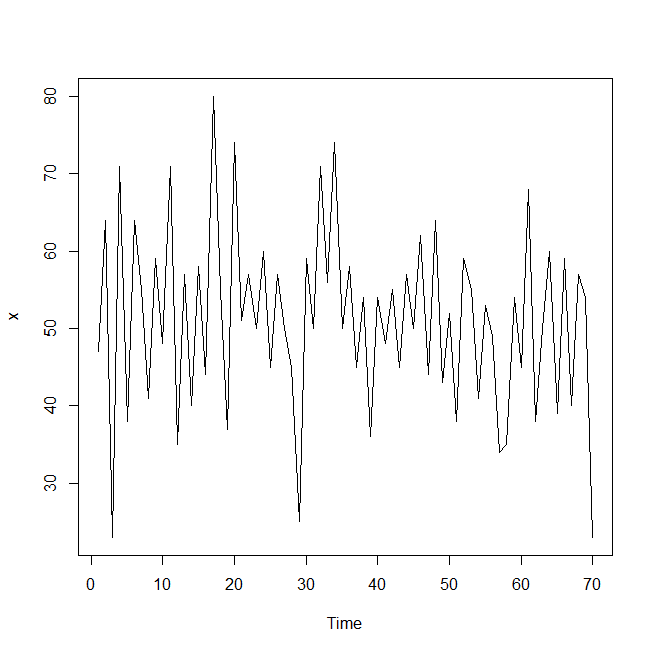时间序列图 序列白噪声检测 for(i in 1:2) print(Box.test(x,lag=6*i)) Box-Pierce test data: x X-squared = 20.209, df = 6, p-value = 0.002542 Box-Pierce test data: x X-squared = 21.622, df = 12, p-value = 0.04198 绘制自相关图和偏自相关图 acf(x) pacf(x)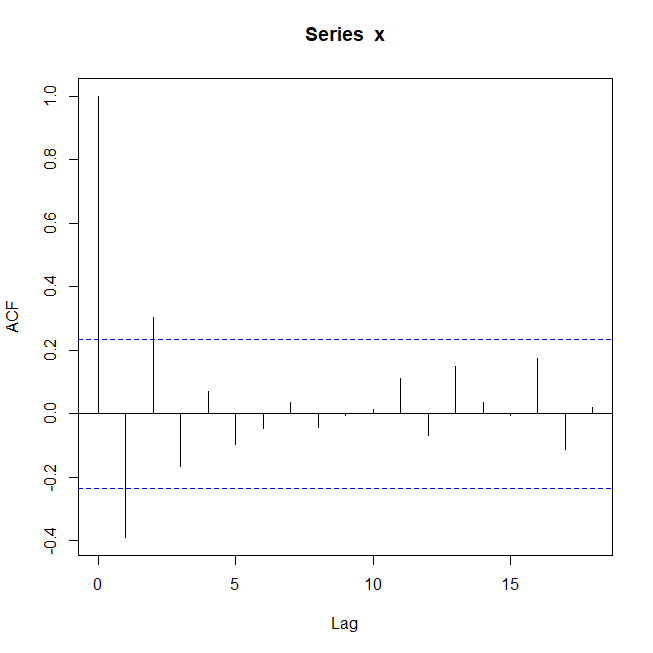ACF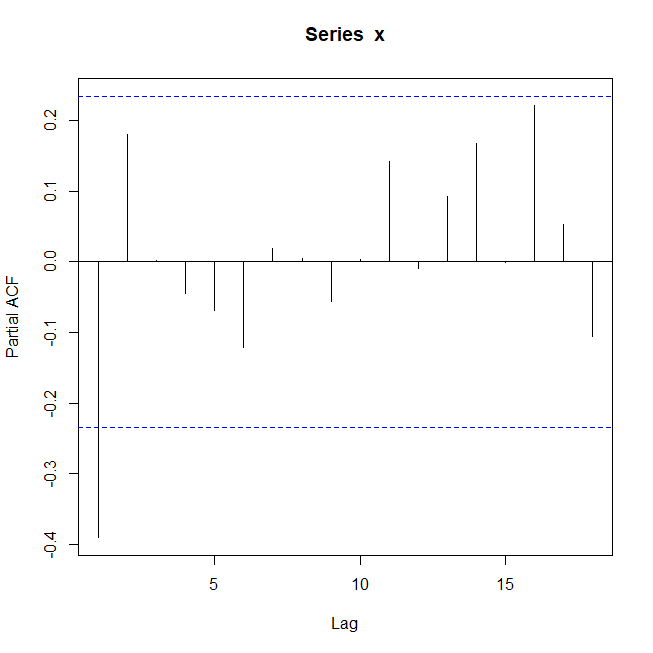PACF 拟合MA(2)模型 > x.fit1<-arima(x,order = c(0,0,2)) > x.fit1 Call: arima(x = x, order = c(0, 0, 2)) Coefficients: ma1 ma2 intercept -0.3194 0.3019 51.1695 s.e. 0.1160 0.1233 1.2516 sigma^2 estimated as 114.4: log likelihood = -265.35, aic = 538.71 MA(2)模型显著性检验 for(i in 1:2) print(Box.test(x.fit1$residual,lag=6*i))

Box-Pierce test

data:  x.fit1$residual X-squared = 2.1105, df = 6, p-value = 0.9093 Box-Pierce test data: x.fit1$residual
X-squared = 3.9217, df = 12, p-value = 0.9848

拟合AR(1)模型

x.fit2<-arima(x,order = c(1,0,0))
> x.fit2

Call:
arima(x = x, order = c(1, 0, 0))

Coefficients:
ar1  intercept
-0.4191    51.2658
s.e.   0.1129     0.9137

sigma^2 estimated as 116.6:  log likelihood = -265.98,  aic = 537.96

#AR(1)模型显著性检验

for(i in 1:2) print(Box.test(x.fit2$residual,lag=6*i)) Box-Pierce test data: x.fit2$residual
X-squared = 4.1678, df = 6, p-value = 0.654

Box-Pierce test

data:  x.fit2\$residual
X-squared = 6.1411, df = 12, p-value = 0.9088

观测可以得知，同一个序列可以构造两个序列模型，两个模型都显著有效，对于如何选择问题，可以引进AIC和SBC(BIC)信息准则的概念进行模型优化。

AIC准则
最小信息量准则，指导思想是拟合模型的优劣可以可以从两个方面进行考虑：一个是衡量拟合程序的似然函数值，模型中未知参数的个数。
但是未知参数越多，说明模型中自变量越多，未知的风险越多，而且参数越多，参数估计的难度就越大，估计的精度也就越差。所以一个好的拟合模型应该是拟合精度和未知参数的个数的综合最优配置。
AIC函数达到最小的模型被认为是最优模型。

另外一种模型

SBC(BIC)准则
AIC模型也有一些不足之处，对于一个观察值序列而言，序列越长，相关信息就越分散，要充分的提取其中的有用信息，或者使拟合精度比较高，通常要包括多个自变量的复杂模型。在AIC准则中拟合误差提供的信息要受到样本容量的放大，而参数个数的惩罚因子却和样本容量没有关系，它的权重始终是不变。
因此当样本无穷大时，由AIC准则选择的模型不收敛于真实模型，它通常比真实模型所含有的未知个数要多。
SBC对AIC的改进就是就是将未知参数个数惩罚权重由常数变成样本容量的对数函数，理论上，SBC的准则确定的最优模型是真实阶数的相合估计。

通过对上例中参数对比：
模型 AIC SBC
MA(2) 538.71 547.7
AR(1) 537.96 544.7
从两个方面比较，AR(1)都要优于MA(2)，AIC准则与SBC准则的提出，可以有效的弥补自相关图与偏自相关图定阶的主观性，在有限有阶数范围内帮助我们找到最优拟合模型。

展开全文时间序列 参数估计 矩估计 最小二乘估计 极大似然估计
• 参数估计

千次阅读 2019-09-02 22:09:01
参数估计包括点估计和区间估计两类。 点估计 点估计是以抽样得到的样本指标作为总体指标的估计量，并以样本指标的实际值直接作为总体未知参数的估计值的一种推断方法。 点估计(point estimate)是用样本统计量的某个...参数估计 统计学 数据分析 区间估计
• Logistic回归简介Logistic回归：主要用于因变量为分类变量(如疾病的缓解、不缓解，评比62616964757a686964616fe78988e69d8331333363383438中的好、中、差等)的回归分析...Odds：称为比值、比数，是某事件发生的可...
• 机器学习中的模型参数估计方法：极大似然估计、贝叶斯估计、最大后验估计。机器学习 参数估计 极大似然估计 贝叶斯估计 最大后验估计
• 文章目录参数估计参数估计的基本原理评价估计量的标准一个总体参数的区间估计两个总体参数估计 参数估计 参数估计是推断统计的重要内容之一。它是在抽样及抽样分布的基础上，根据样本统计量来推断所关心的总体参数...统计学 参数估计
• 数理统计｜参数估计

千次阅读 2018-10-10 23:31:28
一、参数估计内容 1.参数估计的requisites   我默认大家已经掌握了基本的概率论知识，例如了解概率空间、随机变量、概率的含义，会求随机变量的分布函数、数字特征，对基本的大数定理和中心极限定理有一些了解，...参数估计 区间估计 极大似然估计 贝叶斯估计
• 浅谈参数估计

万次阅读 2019-09-03 09:38:34
参数估计是推断统计的重要内容之一,它是在抽样及抽样分布的基础上,根据样本统计量来推断所关心的总体参数. 估计量 如果能够掌握总体的全部数据,那么只需要作一些简单的统计描述,就可以得到所关心的总体特征，比如，...
• 参数估计（点估计和区间估计）

万次阅读 多人点赞 2019-09-06 12:07:06
1.点估计就是用样本统计量来估计总体参数。 概念理解：当我们想知道某一总体的某个指标的情况时，测量整体该指标的数值 的工作量太大，或者不符合实际，这时我们可以采用抽样的方法选取一部分样本测量出他们数值，...参数估计 区间估计
• 推断统计：参数估计和假设检验

千次阅读 多人点赞 2020-03-03 00:35:24
目录 ...  3、参数估计(点估计和区间估计)    1）参数估计、点估计和区间统计的概念    2）点估计说明    3）区间估计说明   4、中心极限定理    1）中心极限定理的概念    2...假设检验 参数估计
• 文章目录参数估计假设检验 参数估计 For 高手： 参数估计（parameter estimation），统计推断的一种。根据从总体中抽取的随机样本来估计总体分布中未知参数的过程。从估计形式看，区分为点估计与区间估计：从构造...机器学习 python 参数估计 假设检验
• 统计推断包括参数估计和假设检验。参数估计就是用样本指标（统计量）来估计总体指标（参数）。 一、参数估计基础-Z分布 在统计应用中，可以把任何一个均数为，标准差为的正态分布转变为,的标准正态分布，即将...参数估计 统计推断 区间估计
• 参数估计与非参数估计

万次阅读 2015-05-06 11:38:58
参数估计（parameter estimation）： 根据从总体中抽取的样本估计总体分布中包含的未知参数的方法。人们常常需要根据手中的数据，分析或推断数据反映的本质规律。即根据样本数据如何选择统计量去推断总体的分布或...
• 最后，我们研究第三个问题，参数估计问题。也即是给定一个观察序列O=O1O2O3...OTO=O_{1}O_{2}O_{3}...O_{T}O=O1​O2​O3​...OT​，如何根据最大似然估计来求模型的参数值？即如何调节模型μ=(S,K,A,B,π)\mu =\left...HMM 参数估计
• 参数估计 已经知道观测数据符合某些模型的概率下，我们可以利用参数估计的方法来确定这些参数值，然后得出概率密度模型。这个过程中用到了一个条件，就是概率分布符合某些模型这个事实。在这个事实上进行加工。 ...
•统计学
• 很难说这些观测的数据符合什么模型，参数估计的方法就失效了，我们只有用非参数估计的办法去估计真实数据符合的概率密度模型了。 因此，本文主要讨论 参数估计和非参数估计问题   1. 参数估计 对我们已经知道观测...
• 注：本文以简单的案例，解释了最大似然估计、最大后验估计以及贝叶斯参数估计的联系和区别。假如你有一个硬币。你把它投掷 3 次，出现了 3 次正面。下一次投掷硬币正面朝上的概率是多少? 这是一个从数据中估计参数的...
• 我们最终想要得到的是一个概率密度的模型，如果我们已经对观测的对象有了一些认识，对观测的现象属于那种类型的概率密度分布已经了解，只是需要确定其中的参数而已，这种情况就是属于参数估...
• 概率论 参数估计与假设检验 区分及例子动机区分概念假设检验基本思想小概率原理原理几种常见假设检验假设检验规则和两类错误检验规则两类错误明确步骤 动机 国内本科教材重计算技巧，轻内在逻辑，大家学完容易忘记。...机器学习 假设检验 参数估计
• 《统计学(第六版)》贾俊平 第7章 参数估计 参数估计 parameter estimation 参数估计就是用样本统计量去估计总体的参数。 估计量 estimator 在参数估计中，用来估计总体参数的统计量称为估计量。样本均值、样本比例、...统计学 参数估计
• 统计狗来总结一下前面各个楼主的回答，先甩答案：logistic回归模型的参数估计问题，是可以用最小二乘方法的思想进行求解的，但和经典的(或者说用在经典线性回归的参数估计问题)最小二乘法不同，是用的是“迭代重加权...
• 在工程物理、 化学工程、 生物医学、 统计学、 经济学、 信号处理、 自动化、测绘学等领域中， 许多问题都可归结为求解矩阵方程 Ax=b的问题，其中最常见的是线性参数估计问题，而最小二乘法是最常用的线性参数估计...
• 1.参数估计 随机变量XXX的分布函数已知，但它的一个或多个参数未知，我们需要根据已有样本，估计XXX分布函数的参数。 2. 点估计 随机变量XXX的分布函数已知，但它的一个或多个参数未知，根据XXX的一个样本估计...参数估计 极大似然估计
• 第 7 章 参数估计 7.1 参数估计的基本原理 参数估计就是用样本统计量去估计总体的参数。比如，用样本均值估计总体均值，用样本比例估计总体比例。 在参数估计中，用来估计总体参数的统计量称为估计量。样本均值、...数理统计 数据分析 机器学习理论基础
• 参数估计:对无偏性的理解

千次阅读 2020-05-26 11:45:39
在学习概率论的"参数估计"一章时有一些概念没能理解清楚，尤其是参数估计量的性质。在反复翻书的过程中总算搞清楚了一些，在这里记录一下我的理解 无偏性 一般书上讲到的第一个性质就是这个，初看很让人头大，如果不...数学
• 有监督参数估计已知分类器结构或函数形式，从训练样本中估计参数。 本文主要介绍贝叶斯决策（详见 贝叶斯决策的过程 ）条件概率密度的有监督参数估计过程。方法有最大似然估计和贝叶斯参数估计法。 最大似然...最大似然估计 贝叶斯估计 贝叶斯分类
• 衡量参数估计的指标2.1 无偏性2.2 一致性2.3 有效性3. 一些引理3.1 期望运算的线性性3.2 期望运算的线性性4. *β*^~OLS~ 的性质4.1 *β*^~OLS~ 服从的分布4.2 *β*^~OLS~ 与误差项之间的关系4.3 *β*^~OLS~ 的无偏...统计学 机器学习...